# Chapter # 08 : Introduction to Graph | Depth-First-Search(DFS) | Breadth-First-Search(BFS)

### Introduction to Graph

.

A graph is a pictorial representation of a set of objects where some pairs of objects are connected by links. The interconnected objects are represented by points termed as vertices, and the links that connect the vertices are called edges.

Formally, a graph is a pair of sets (V, E), where V is the set of vertices and Eis the set of edges, connecting the pairs of vertices. Take a look at the following graph.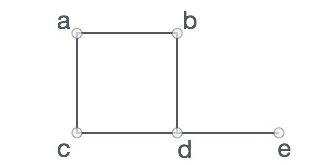Figure 1: Graph

In the above graph,

V = {a, b, c, d, e}

E = {ab, ac, bd, cd, de}

## Graph Data Structure

Mathematical graphs can be represented in data structure. We can represent a graph using an array of vertices and a two-dimensional array of edges. Before we proceed further, let's familiarize ourselves with some important terms −

• Vertex − Each node of the graph is represented as a vertex. In the following example, the labeled circle represents vertices. Thus, A to G are vertices. We can represent them using an array as shown in the following image. Here A can be identified by index 0. B can be identified using index 1 and so on.

• Edge − Edge represents a path between two vertices or a line between two vertices. In the following example, the lines from A to B, B to C, and so on represents edges. We can use a two-dimensional array to represent an array as shown in the following image. Here AB can be represented as 1 at row 0, column 1, BC as 1 at row 1, column 2 and so on, keeping other combinations as 0.

• Adjacency − Two node or vertices are adjacent if they are connected to each other through an edge. In the following example, B is adjacent to A, C is adjacent to B, and so on.

• Path − Path represents a sequence of edges between the two vertices. In the following example, ABCD represents a path from A to D.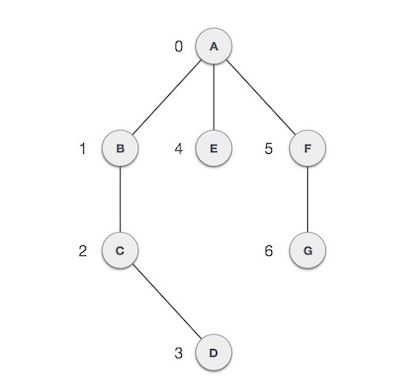Figure 2: Sample Graph

### Depth-First-Search (DFS)

Depth First Search (DFS) algorithm traverses a graph in a depth ward motion and uses a stack to remember to get the next vertex to start a search, when a dead end occurs in any iteration.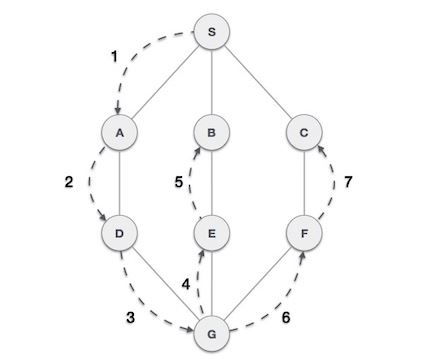Figure 3: DFS

As in the example given above, DFS algorithm traverses from S to A to D to G to E to B first, then to F and lastly to C. It employs the following rules.

• Rule 1 − Visit the adjacent unvisited vertex. Mark it as visited. Display it. Push it in a stack.

• Rule 2 − If no adjacent vertex is found, pop up a vertex from the stack. (It will pop up all the vertices from the stack, which do not have adjacent vertices.)

• Rule 3 − Repeat Rule 1 and Rule 2 until the stack is empty.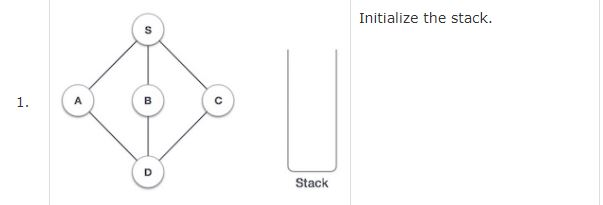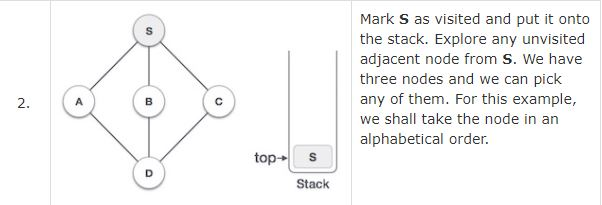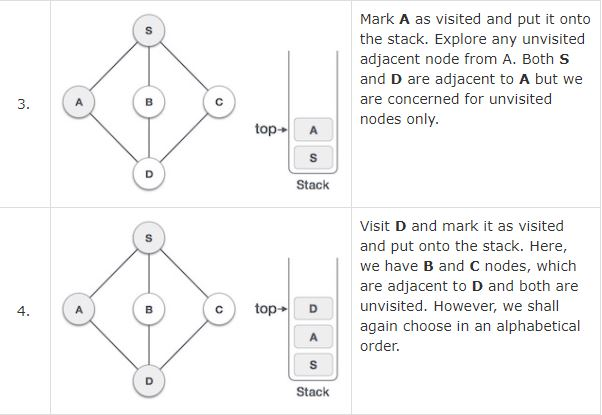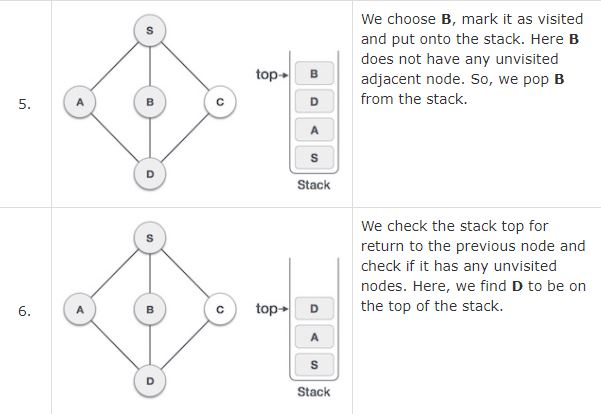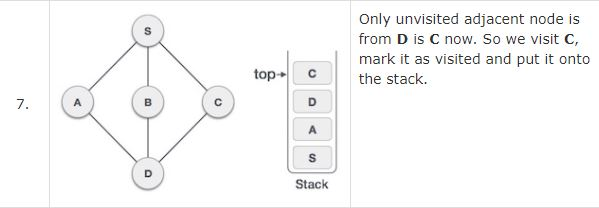As C does not have any unvisited adjacent node so we keep popping the stack until we find a node that has an unvisited adjacent node. In this case, there's none and we keep popping until the stack is empty.

Breadth First Search (BFS) algorithm traverses a graph in a breadth ward motion and uses a queue to remember to get the next vertex to start a search, when a dead end occurs in any iteration.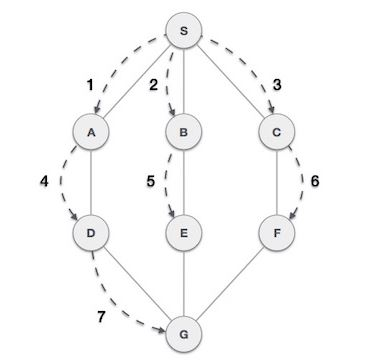Figure 4: BFS

As in the example given above, BFS algorithm traverses from A to B to E to F first then to C and G lastly to D. It employs the following rules.

• Rule 1 − Visit the adjacent unvisited vertex. Mark it as visited. Display it. Insert it in a queue.

• Rule 2 − If no adjacent vertex is found, remove the first vertex from the queue.

• Rule 3 − Repeat Rule 1 and Rule 2 until the queue is empty.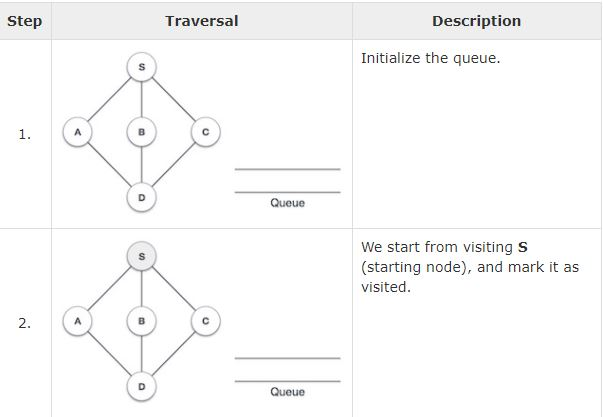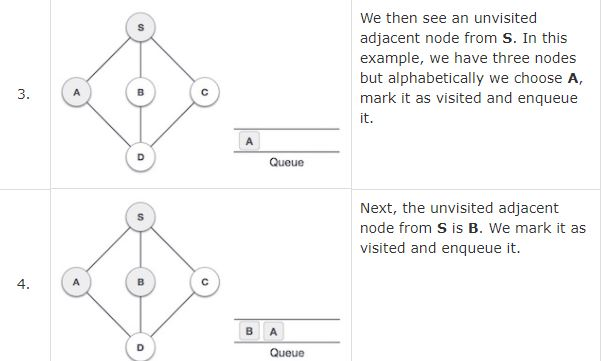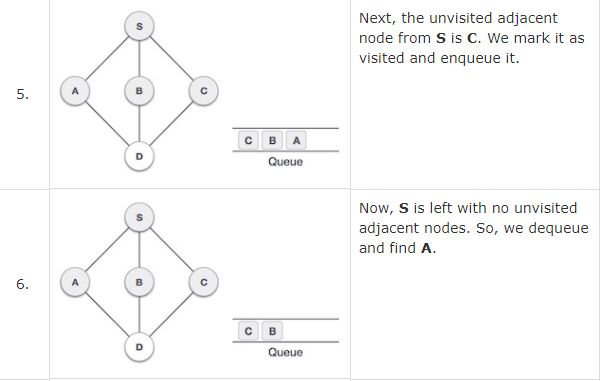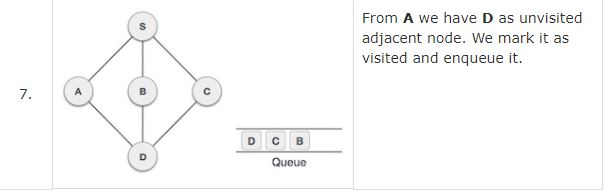At this stage, we are left with no unmarked (unvisited) nodes. But as per the algorithm we keep on de-queuing in order to get all unvisited nodes. When the queue gets emptied, the program is over.